## Example Questions

← Previous 1

### Example Question #1 : How To Find The Slope Of A Perpendicular Line

What is the slope of a line perpendicular to line passing through the points (5, 7) and (2, 12)?

5/3

5/7

3/5

–7/5

–3/5

3/5

Explanation:

We find the slope of a perpendicular line by taking the opposite and reciprocal of our given line's slope; therefore, we must first solve for the slope of our line. Given our points, we must find the ratio of the rise to the run, that is:

slope = rise/run = (y2 – y1)/(x2 – x1)

slope = (7 – 12)/(5 – 2) = –5/3

The perpendicular will be "flipped" (i.e. reciprocal) and also positive (opposite); therefore, it is: 3/5.

### Example Question #81 : Lines

Which of the following lines has a slope perpendicular to the line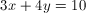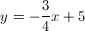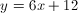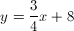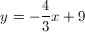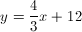Explanation:

The slope of the original equation is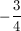. (This can be found by transforming into the form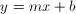, yielding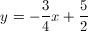.

Thus, the slope of any perpendicular line will be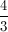### Example Question #3 : How To Find The Slope Of A Perpendicular Line

A line in the x-y plane passes through the points (3,5) and (–2, 4). Another line does not pass through the origin, and does not have the same intercept as the first line. If the two lines are perpendicular, what is the slope of the second line?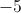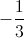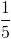Explanation:

Disregard the information about the second line not passig through the origin and not having a particular intercept—it is largely irrelevant.

The important information is the fact that the second line, as mentioned, is perpendicular to the first. This means that its slope is the negative reciprocal of the slope of the first line.

We can find the slope of the first line using the slope formula and our two points, (3,5) and (–2, 4).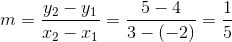The slope of the second line is the negative reciprocal of, meaning it must be.

### Example Question #4 : How To Find The Slope Of A Perpendicular Line

Which of the following represents a line that is perpendicular to the equation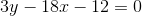?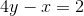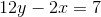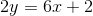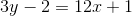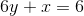Explanation:

Begin by putting the original equation into the form of: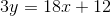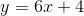To find a line that is perpendicular to the above, take the slope, find the reciprocal, and change the sign on it. In other words, since our slope is 6, a line that is perpendicular will have an-value of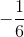.

Now go through each answer choice and put it into the form of. The correct answer will be the one with an-value of.

In this case, it will be the equation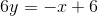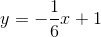### Example Question #1 : How To Find The Slope Of A Perpendicular Line

What is the slope of any line perpendicular to 2y = 4x +3 ?

– ½

½

– 4

2

– ½

Explanation:

First, we must solve the equation for y to determine the slope:  y = 2x + 3/2

By looking at the coefficient in front of x, we know that the slope of this line has a value of 2. To fine the slope of any line perpendicular to this one, we take the negative reciprocal of it:

slope = m , perpendicular slope = – 1/m

slope = 2 , perpendicular slope = – 1/2

### Example Question #1 : How To Find The Slope Of A Perpendicular Line

What line is perpendicular to 2x + y = 3 at (1,1)?

3x + 2y = 1

x – 2y = -1

2x + 3y = 1

x + 2y - 3

x – 2y = -1

Explanation:

Find the slope of the given line.  The perpendicular slope will be the opposite reciprocal of the original slope.  Use the slope-intercept form (y = mx + b) and substitute in the given point and the new slope to find the intercept, b.  Convert back to standard form of an equation:  ax + by =  c.

### Example Question #1 : How To Find The Slope Of A Perpendicular Line

What is the slope of the line perpendicular to the line given by the equation

6x – 9y +14 = 0

2/3

-2/3

6

-1/6

-3/2

-3/2

Explanation:

First rearrange the equation so that it is in slope-intercept form, resulting in y=2/3 x + 14/9. The slope of this line is 2/3, so the slope of the line perpendicular will have the opposite reciprocal as a slope, which is -3/2.

### Example Question #1 : How To Find The Slope Of A Perpendicular Line

What is the slope of the line perpendicular to the line represented by the equation y = -2x+3?

1/2

-1/2

2

-2/3

2/3

1/2

Explanation:

Perpendicular lines have slopes that are the opposite of the reciprocal of each other. In this case, the slope of the first line is -2. The reciprocal of -2 is -1/2, so the opposite of the reciprocal is therefore 1/2.

### Example Question #1 : How To Find The Slope Of A Perpendicular Line

Find the slope of a line perpendicular to the line y = –3x – 4.

4

1/4

–3

1/3

1/3

Explanation:

First we must find the slope of the given line. The slope of y = –3x – 4 is –3. The slope of the perpendicular line is the negative reciprocal. This means you change the sign of the slope to its opposite: in this case to 3. Then find the reciprocal by switching the denominator and numerator to get 1/3; therefore the slope of the perpendicular line is 1/3.

### Example Question #6 : How To Find The Slope Of A Perpendicular Line

What is the slope of a line perpendicular to the following: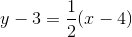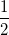Explanation:

The question puts the line in point-slope form y – y1 = m(x  x1), where m is the slope. Therefore, the slope of the original line is 1/2.  A line perpendicular to another has a slope that is the negative reciprocal of the slope of the other line. The negative reciprocal of the original line is 2, and is thus the slope of its perpendicular line.

← Previous 1

Tired of practice problems?

Try live online GRE Math prep today.